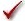## Please enable JavaScript to view this site.

 Navigation: PRINCIPLES OF STATISTICS > Analysis checklists Analysis checklist: Mann-Whitney test

The Mann-Whitney test is a nonparametric test that compares the distributions of two unmatched groups. It is sometimes said to compare medians, but this is not always true.

###Are the “errors” independent?

The term “error” refers to the difference between each value and the group median. The results of a Mann-Whitney test only make sense when the scatter is random – that whatever factor caused a value to be too high or too low affects only that one value. Prism cannot test this assumption. You must think about the experimental design. For example, the errors are not independent if you have six values in each group, but these were obtained from two animals in each group (in triplicate). In this case, some factor may cause all triplicates from one animal to be high or low.

###Are the data unpaired?

The Mann-Whitney test works by ranking all the values from low to high, and comparing the mean rank in the two groups. If the data are paired or matched, then you should choose a Wilcoxon matched pairs test instead.

###Are you comparing exactly two groups?

Use the Mann-Whitney test only to compare two groups. To compare three or more groups, use the Kruskal-Wallis test followed by post tests. It is not appropriate to perform several Mann-Whitney (or t) tests, comparing two groups at a time.

###Do the two groups follow data distributions with the same shape?

If the two groups have distributions with similar shapes, then you can interpret the Mann-Whitney test as comparing medians. If the distributions have different shapes, you really cannot interpret the results of the Mann-Whitney test.

###Do you really want to compare medians?

The Mann-Whitney test compares the medians of two groups (well, not exactly). It is possible to have a tiny P value – clear evidence that the population medians are different – even if the two distributions overlap considerably.

###If you chose a one-tail P value, did you predict correctly?

If you chose a one-tail P value, you should have predicted which group would have the larger median before collecting any data. Prism does not ask you to record this prediction, but assumes that it is correct. If your prediction was wrong, then ignore the P value reported by Prism and state that P>0.50. One- vs. two-tail P values.

###Are the data sampled from non-Gaussian populations?

By selecting a nonparametric test, you have avoided assuming that the data were sampled from Gaussian distributions, but there are drawbacks to using a nonparametric test. If the populations really are Gaussian, the nonparametric tests have less power (are less likely to give you a small P value), and that difference is quite noticeable with small sample sizes.